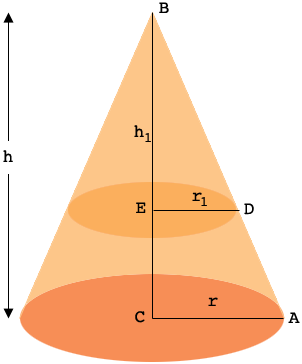SEARCH HOMEMath Central Quandaries & QueriesQuestion from Danica: What portion of the height from top that the cone be cut into two equal volume?Hi Danica,

I can help get you started.

In my diagram the large cone has radius $r$ and height $h$ and the top part of the cone has radius $r_1$ and height $h_{1}.$ Your task is to determine the fraction $f = \large \frac{h_1}{h}.$The triangles $ABC$ and $DBE$ are similar so

$\frac{r_1}{r} = \frac{h_1}{h} = f.$

Write back if you need more assistance,
PennyMath Central is supported by the University of Regina and The Pacific Institute for the Mathematical Sciences.